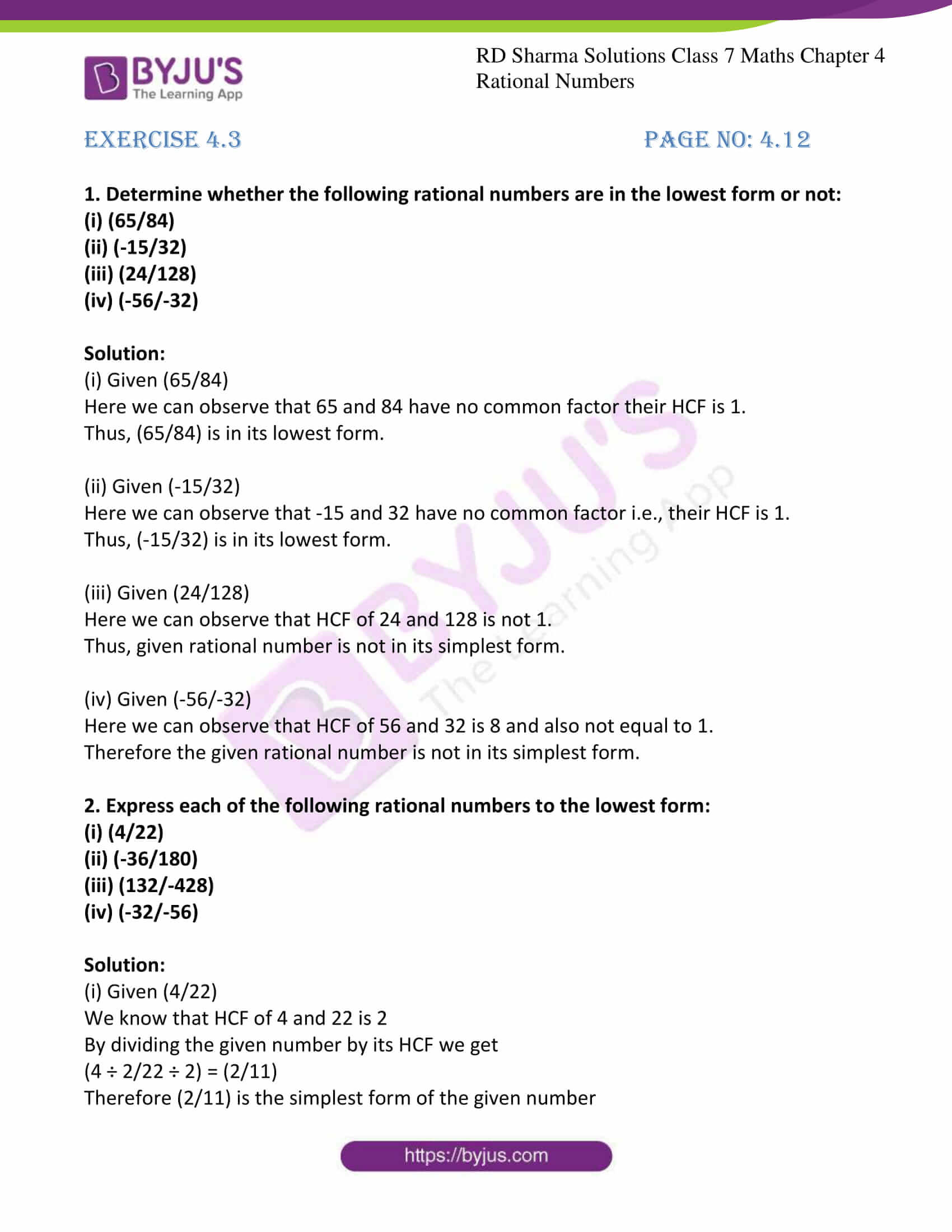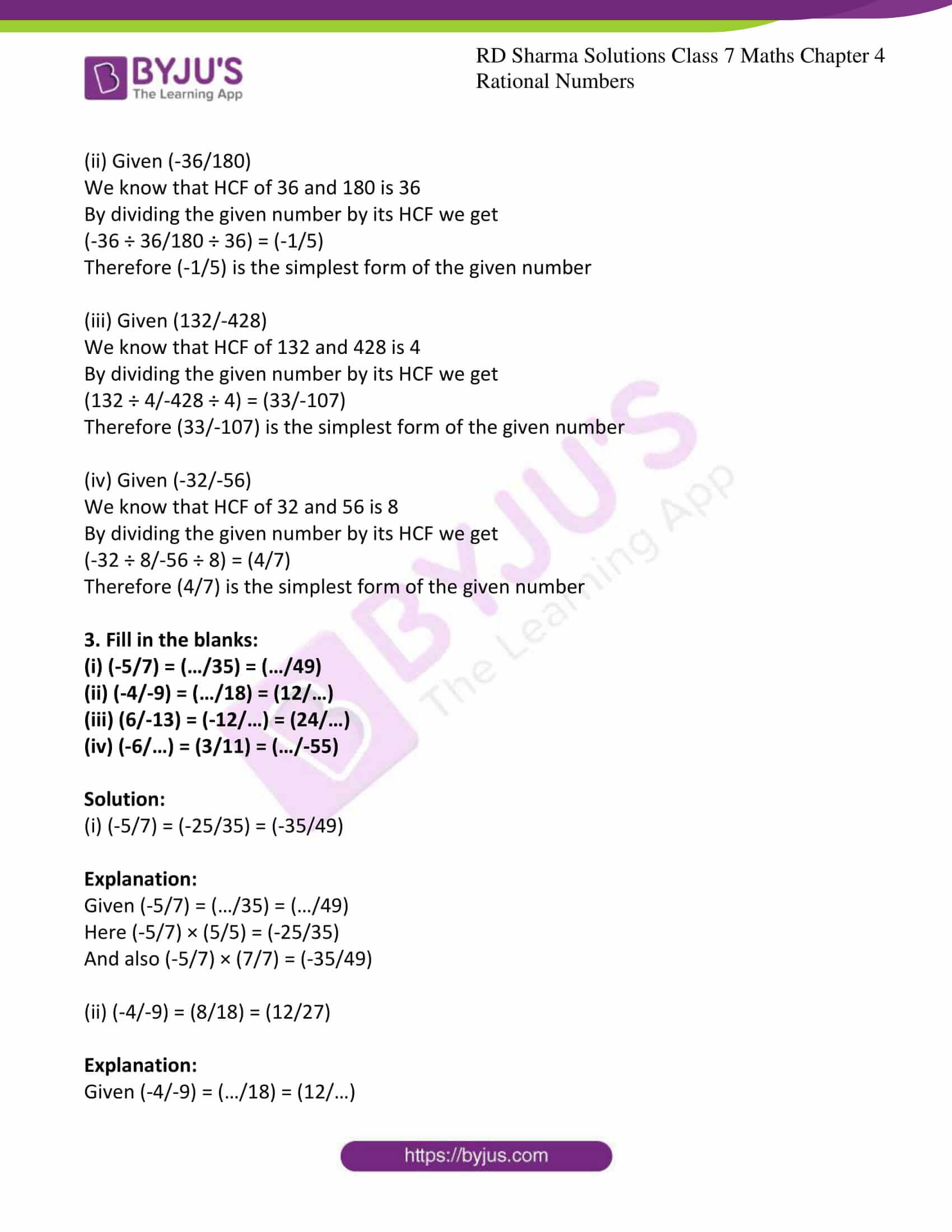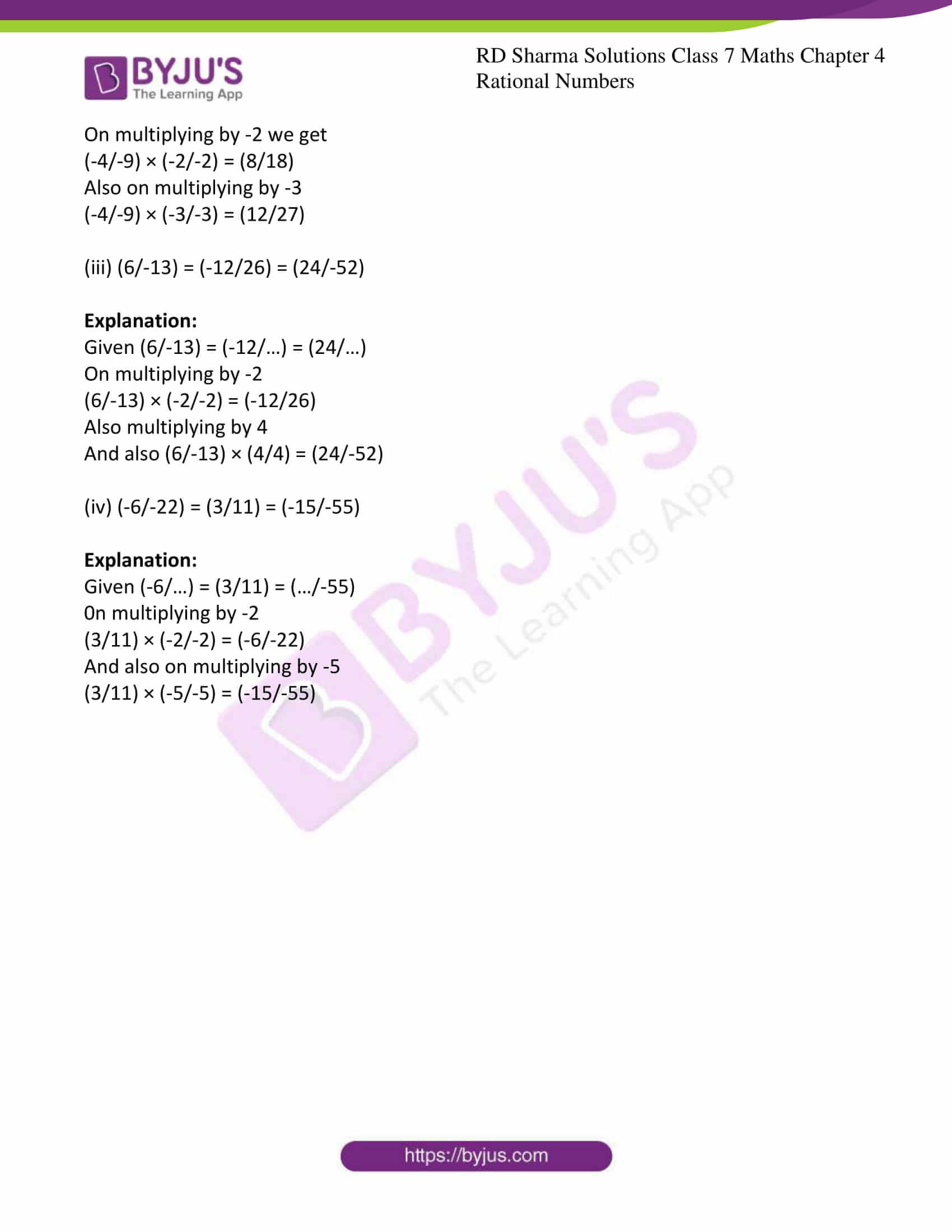# RD Sharma Solutions For Class 7 Maths Exercise 4.3 Chapter 4 Rational Numbers

Students can refer and download the RD Sharma Solutions for Class 7 Maths Exercise 4.3 of Chapter 4 from the links provided below. The syllabus given by the CBSE for all the classes is completely based on the RD Sharma textbook. Hence, preparation of exams using the RD Sharma Class 7 will definitely provide students with an advantage of scoring well. This exercise of RD Sharma Solutions of chapter 4 contains 3 questions based on the lowest form of rational numbers. A rational number p/q is said to be in the lowest form if p and q have no common factor other than 1. To know more about rational numbers by solving RD Sharma Solutions for class 7 along with the solutions provided here.

## Download the PDF of RD Sharma Solutions For Class 7 Maths Chapter 4 – Rational Numbers Exercise 4.3### Access answers to Maths RD Sharma Solutions For Class 7 Chapter 4 – Rational Numbers Exercise 4.3

1. Determine whether the following rational numbers are in the lowest form or not:

(i) (65/84)

(ii) (-15/32)

(iii) (24/128)

(iv) (-56/-32)

Solution:

(i) Given (65/84)

Here we can observe that 65 and 84 have no common factor their HCF is 1.

Thus, (65/84) is in its lowest form.

(ii) Given (-15/32)

Here we can observe that -15 and 32 have no common factor i.e., their HCF is 1.

Thus, (-15/32) is in its lowest form.

(iii) Given (24/128)

Here we can observe that HCF of 24 and 128 is not 1.

Thus, given rational number is not in its simplest form.

(iv) Given (-56/-32)

Here we can observe that HCF of 56 and 32 is 8 and also not equal to 1.

Therefore the given rational number is not in its simplest form.

2. Express each of the following rational numbers to the lowest form:

(i) (4/22)

(ii) (-36/180)

(iii) (132/-428)

(iv) (-32/-56)

Solution:

(i) Given (4/22)

We know that HCF of 4 and 22 is 2

By dividing the given number by its HCF we get

(4 ÷ 2/22 ÷ 2) = (2/11)

Therefore (2/11) is the simplest form of the given number

(ii) Given (-36/180)

We know that HCF of 36 and 180 is 36

By dividing the given number by its HCF we get

(-36 ÷ 36/180 ÷ 36) = (-1/5)

Therefore (-1/5) is the simplest form of the given number

(iii) Given (132/-428)

We know that HCF of 132 and 428 is 4

By dividing the given number by its HCF we get

(132 ÷ 4/-428 ÷ 4) = (33/-107)

Therefore (33/-107) is the simplest form of the given number

(iv) Given (-32/-56)

We know that HCF of 32 and 56 is 8

By dividing the given number by its HCF we get

(-32 ÷ 8/-56 ÷ 8) = (4/7)

Therefore (4/7) is the simplest form of the given number

3. Fill in the blanks:

(i) (-5/7) = (…/35) = (…/49)

(ii) (-4/-9) = (…/18) = (12/…)

(iii) (6/-13) = (-12/…) = (24/…)

(iv) (-6/…) = (3/11) = (…/-55)

Solution:

(i) (-5/7) = (-25/35) = (-35/49)

Explanation:

Given (-5/7) = (…/35) = (…/49)

Here (-5/7) × (5/5) = (-25/35)

And also (-5/7) × (7/7) = (-35/49)

(ii) (-4/-9) = (8/18) = (12/27)

Explanation:

Given (-4/-9) = (…/18) = (12/…)

On multiplying by -2 we get

(-4/-9) × (-2/-2) = (8/18)

Also on multiplying by -3

(-4/-9) × (-3/-3) = (12/27)

(iii) (6/-13) = (-12/26) = (24/-52)

Explanation:

Given (6/-13) = (-12/…) = (24/…)

On multiplying by -2

(6/-13) × (-2/-2) = (-12/26)

Also multiplying by 4

And also (6/-13) × (4/4) = (24/-52)

(iv) (-6/-22) = (3/11) = (-15/-55)

Explanation:

Given (-6/…) = (3/11) = (…/-55)

0n multiplying by -2

(3/11) × (-2/-2) = (-6/-22)

And also on multiplying by -5

(3/11) × (-5/-5) = (-15/-55)